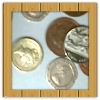#### You may also like### Coin Tossing Games

You and I play a game involving successive throws of a fair coin. Suppose I pick HH and you pick TH. The coin is thrown repeatedly until we see either two heads in a row (I win) or a tail followed by a head (you win). What is the probability that you win?### Win or Lose?

A gambler bets half the money in his pocket on the toss of a coin, winning an equal amount for a head and losing his money if the result is a tail. After 2n plays he has won exactly n times. Has he more money than he started with?A counter is placed in the bottom right hand corner of a grid. You toss a coin and move the star according to the following rules: ... What is the probability that you end up in the top left-hand corner of the grid?

# Who's the Winner?

##### Age 14 to 16Challenge Level

Thank you to Anna from Mt Eliza North Primary School, Australia, who sent us this solution:

1. What are the possible results if 2 goals are scored in total?

2-0, 0-2, 1-1.

2. Why are they not all equally likely?

They are not all equally likely because there are two ways you can get the end result, 1-1, by the teachers scoring first and then the students or the students scoring first and then the teachers. There is one way for the end result, 2-0, the teachers get the 2 goals without the students getting 1. And there is only one way you can get the end result 0-2, by the students getting both the goals. Therefore the end result 1-1 is more likely.

3. Is this a reasonable assumption?

Yes. Even if the teachers are more confident and the students are trying harder, those equal out so the chance would stay at 50-50.

4. What are the probabilities of each result according to Alison's model?

In Alison's model the team who scores first is twice as likely to score the next goal.

The chance of ending 2-0, with the teachers winning, is:

$\frac{1}{2}$$\times$$\frac{2}{3}$$=$$\frac{1}{3}$

so there would be a 33.3% chance of that occurring.

The chance of ending 2-0, with the students winning, is also 33.3%:

$\frac{1}{2}$$\times$$\frac{2}{3}$$=$$\frac{1}{3}$

The chance of ending 1-1, with the teachers scoring the first goal and the students scoring the second goal, is:

$\frac{1}{2}$$\times$$\frac{1}{3}$$=$$\frac{1}{6}$

The chance of ending 1-1, with the students scoring the first goal and the teachers scoring the second goal, is also:

$\frac{1}{2}$$\times$$\frac{1}{3}$$=$$\frac{1}{6}$

So altogether the chance of ending 1-1 is also 33.3%:

$\frac{1}{6}$+$\frac{1}{6}$=$\frac{1}{3}$

Therefore, using Alison's model there is a 33.3% chance of any of the three results occurring.

5. What are the probabilities of each result according to Charlie's model?

In Charlie's model, after a team scores, the opposing team are twice as likely to score the next goal, because they start trying harder.

The chance of ending 2-0, with the teachers winning, is:

$\frac{1}{2}$$\times$$\frac{1}{3}$$=$$\frac{1}{6}$

so there would be a 16.7% chance of that occurring.

The chance of ending 2-0, with the teachers winning, is also 16.7%:

$\frac{1}{2}$$\times$$\frac{1}{3}$$=$$\frac{1}{6}$

The chance of ending 1-1, with the teachers scoring the first goal and the students scoring the second goal, is:

$\frac{1}{2}$$\times$$\frac{2}{3}$$=$$\frac{1}{3}$

The chance of ending 1-1, with the students scoring the first goal and the teachers scoring the second goal, is also:

$\frac{1}{2}$$\times$$\frac{2}{3}$$=$$\frac{1}{3}$

So altogether the chance of ending 1-1 is 66.6%:

$\frac{1}{3}$+$\frac{1}{3}$=$\frac{2}{3}$

Therefore, using Charlie's model there is a much greater chance of ending up with a 1-1 result.

Krystof from Uhelny Trh, Prague, used tree diagrams to work out the probabilities.